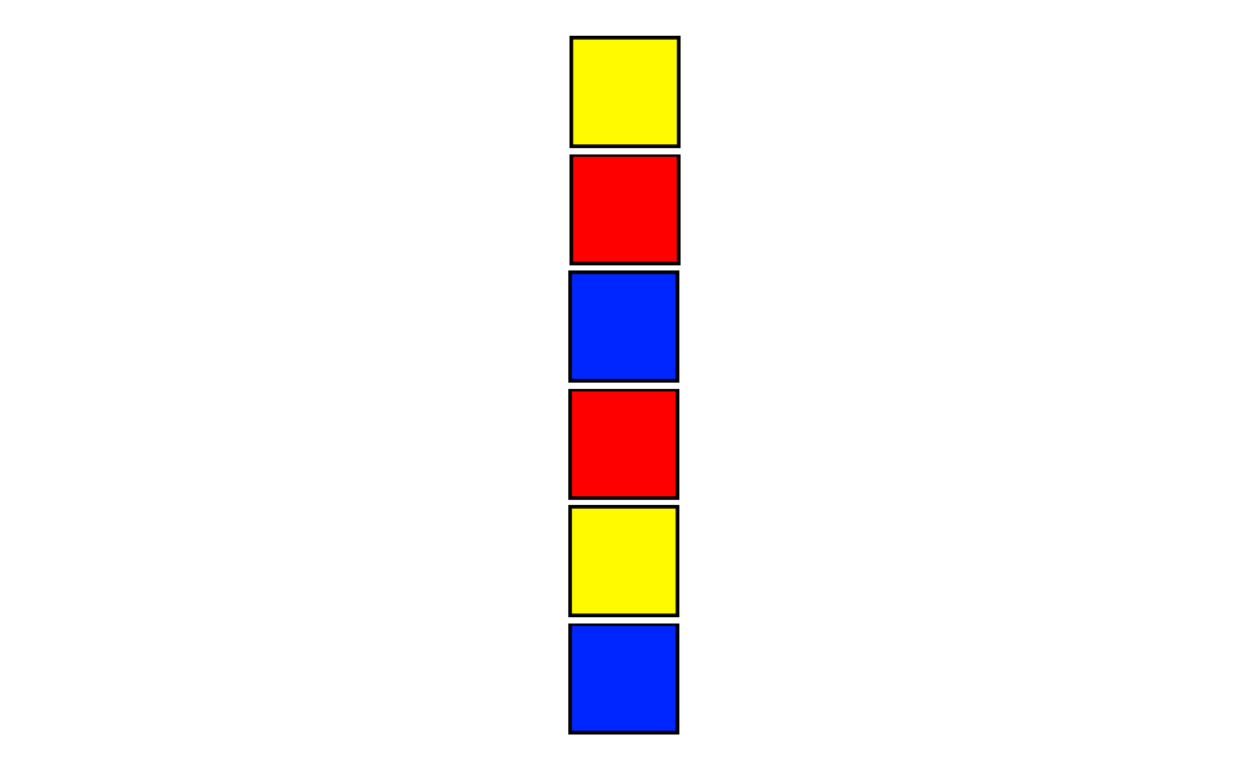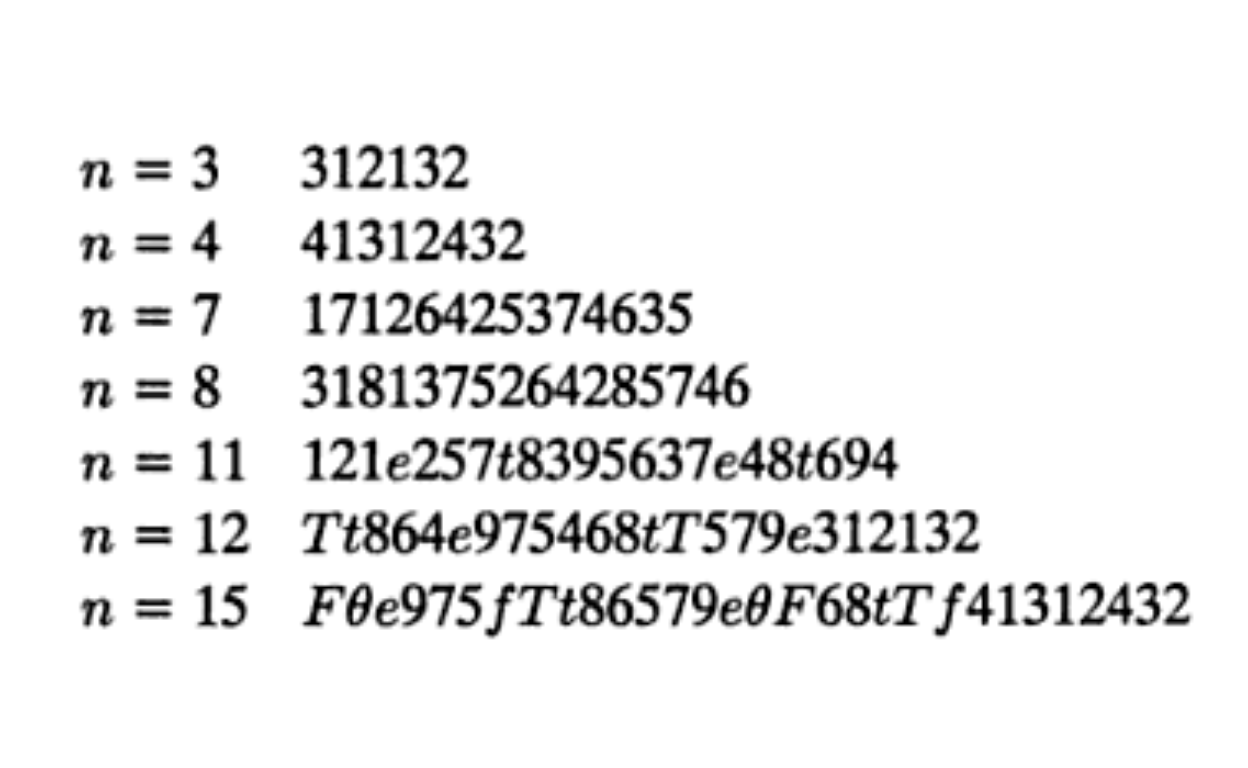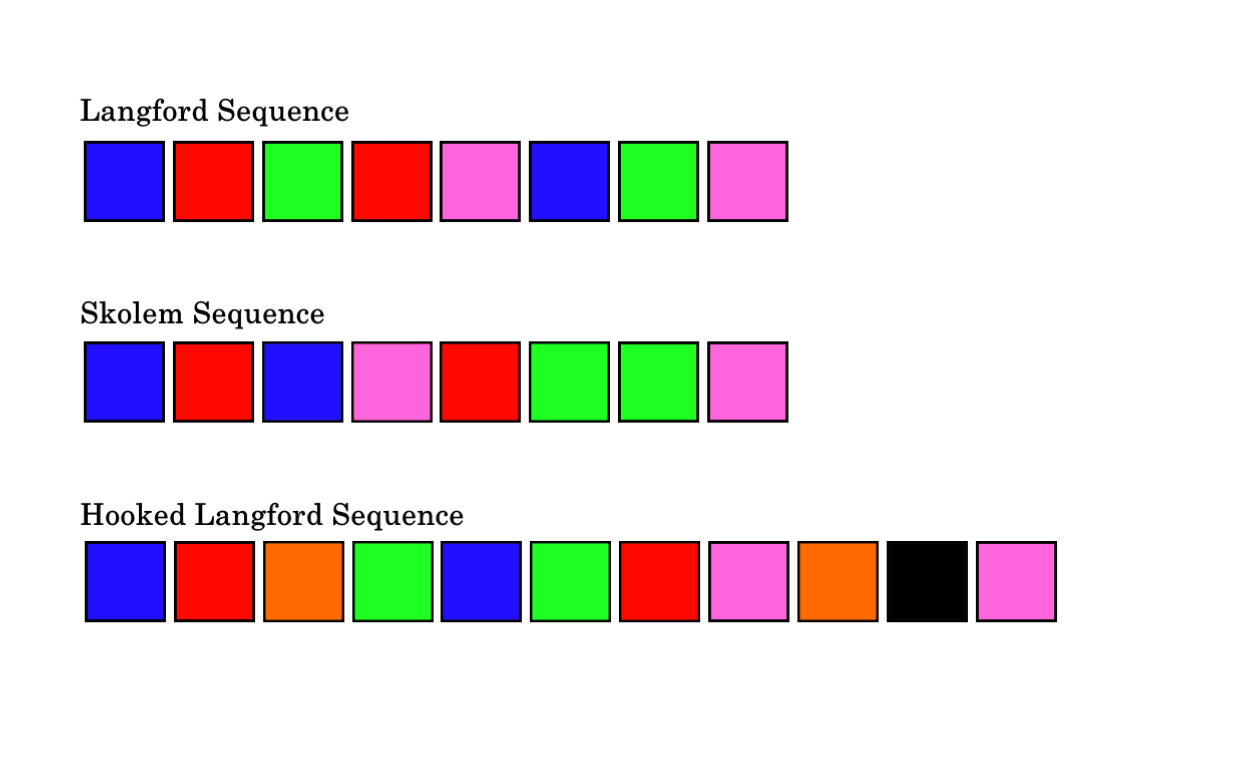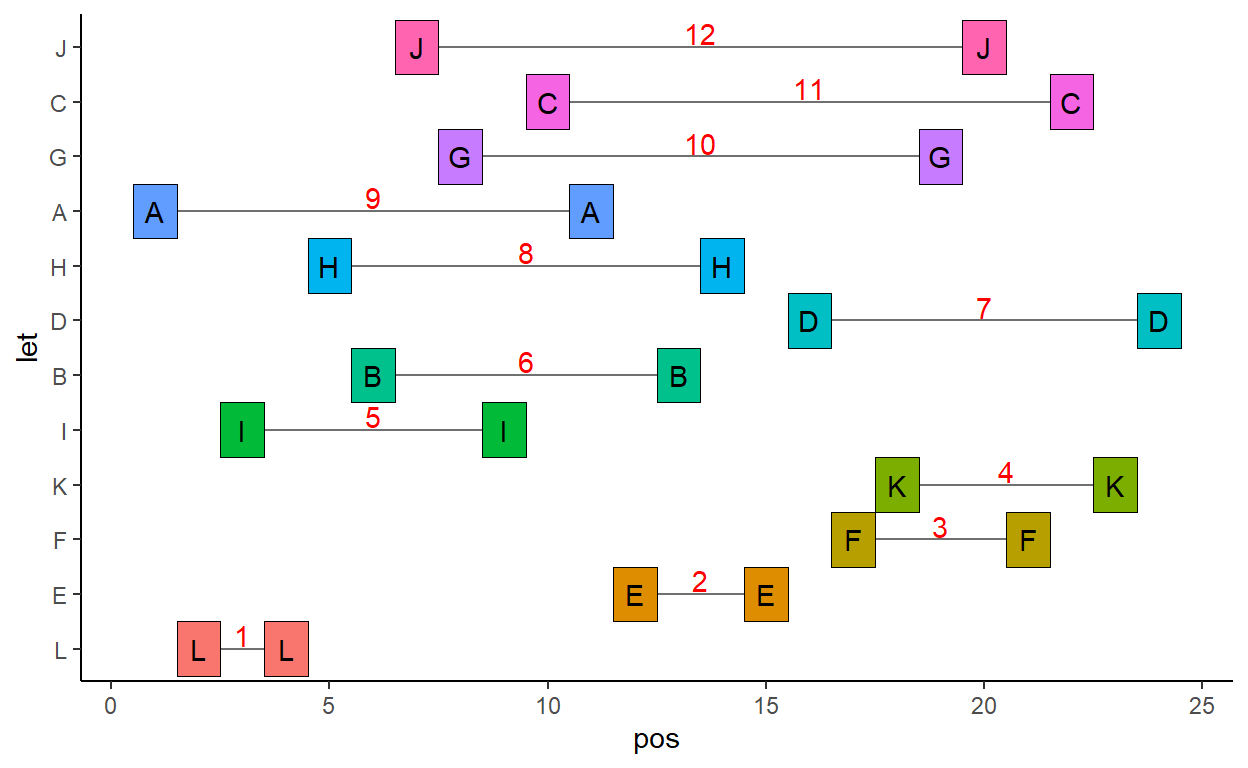# Langford Sequences

Describing Langford and Skolem sequences and how to search for them in R.

James Curley jamescurley.blog
10-16-2020

In 1958, the mathematician C.D. Langford was watching his son play with blocks. He wrote:

There were two of each color, and one day I noticed that he had placed them in a single pile so that between the red pair there was one block, two between the blue pair, and three between the yellow. I then found that by a complete rearrangement I could add a green pair with four between them.The general problem was summarized by Hayasaka & Saito (1979) as: “Given $$2n$$ numbers, two each of the numbers $$1, 2, …., n$$ to find whether they can be arranged in a row in such a way that the two occurrences of each number $$k$$ are separated by exactly $$k$$ other elements of the row.

Langford actually discovered several examples for various different number of blocks. Here are some examples of his sequences:What one might notice is that all the solutions are for a number of blocks $$n$$ that are divisible by 4, or one less than a number that is divisible by 4. A bit more explanation for why this is so can be found at Nick Berry’s excellent blog.

As $$n$$ increases, there are an increasing number of Langford Sequences. There is only one solution for $$n=3$$ & $$n=4$$, 26 solutions for $$n=7$$, 150 for $$n=8$$, 17,792 for $$n=11$$, 108,144 for $$n=12$$ ….. 46,845,158,056,515,936 for $$n=24$$ ! Obviously, trying to find all solutions for any given $$n$$ is computationally intensive!

Although Langford sequences can only be found when $$n$$ is divisible by 4 or when $$n+1$$ is divisible by 4, there is an alternative type of Langford sequence called a Hooked Langford Sequence which can be found for all values of $$n$$. A Hooked Langford Sequence is one where another block/letter is inserted in the penultimate position in the sequence.

At the same time as Langford wrote his piece, a related phenomenon was being independently researched by a Swedish mathematician, Thoralf Skolem, who was trying to form sequences of $$2n$$ blocks with intervals of $$0,1,2,3 ... n$$.

My aim here is to write a small script that can find these sequences. Below is an image of each type of these sequences:### Langford’s Sequences

Say we have $$2*8$$ blocks, let’s represent them with letters. We can then generate a randomly sample sequence of these letters as a starting point. A random shuffle is unlikely to be a Langford sequence. We an check by finding the differences between the positions/indexes of each letter in the vector:


x <- rep(LETTERS[1:8],2)
set.seed(1)
y <- sample(x)
y

 "E" "F" "A" "D" "C" "B" "C" "G" "F" "A" "B" "G" "E" "H" "D" "H"

This gets the difference (number of letters between) the pairs of A’s:


abs(diff(which(y=="A")))-1  #6

 6

Likewise for the D’s and the H’s:


abs(diff(which(y=="D")))-1  #10

 10

abs(diff(which(y=="H")))-1  #1

 1

We can get the results for all the individual letters with a loop:


res<-NULL
for(i in 1:8){  res[[i]] <- abs(diff(which(y==LETTERS[i])))-1 }

names(res)<-LETTERS[1:8]
res

A  B  C  D  E  F  G  H
6  4  1 10 11  6  3  1 

This is fine for one randomly sampled sequence, but when finding Langford sequences, we might want to check many sequences, so it is better to have a faster function. match() seems to be pretty fast for this purpose:


langford <- function(x) {
ux = unique(x)
mux = match(x, ux)
ans = integer(length(ux))
for(i in seq_along(x)) ans[mux[i]] = i - ans[mux[i]]
return(setNames(ans - 1L, ux))
}

langford(y)

E  F  A  D  C  B  G  H
11  6  6 10  1  4  3  1 

### Finding a Langford Sequence

Say we wanted to find a solution for 8 blocks. My strategy here is to keep sampling sequences until one satisfies the criteria. The way to check whether a sequence is a Langford sequence is to sort the intervals between each pair of letters and then ask if this is equal to 1:8. If it is, then we break and return that sequence.


set.seed(17)
while(TRUE) {

samps <- sample(x)
tmp <- sort(langford(samps))

if(sum(tmp==c(1:8))==8)

break
}

samps

 "G" "F" "D" "A" "E" "H" "A" "D" "F" "G" "E" "C" "B" "H" "B" "C"

We can see that this is a Langford Sequence:


sort(langford(samps))  ## the interval between pairs of letters in the sequence

B A C D E F H G
1 2 3 4 5 6 7 8 

This is obviously a brute force approach, and not a cute algorithm that optimally finds solutions. We can generalize for any ‘n’:


get.langford <- function(n){

while(TRUE) {

x <- rep(LETTERS[1:n],2)
samps <- sample(x)
tmp <- sort(langford(samps))

if(sum(tmp==c(1:n))==n)

break
}

return(samps)
}

I could add some if-stop ‘s to this to prevent someone from entering a $$n$$ that will not work (e.g. 5,6). Also, if someone wanted to use an $$n$$ of >26 then we’d have to use combinations of letters. I don’t recommend this though, as the function is pretty slow for anything greater than $$n=12$$.

I think the strategy could also be optimized such that rather than randomly sampling sequences, the sequences tested are done so in a more systematic fashion.

Nevertheless, here it is working for $$n=12$$.


set.seed(77)
lang12 <- get.langford(12)
lang12

 "A" "L" "I" "L" "H" "B" "J" "G" "I" "C" "A" "E" "B" "H" "E" "D"
 "F" "K" "G" "J" "F" "C" "K" "D"


sort(langford(lang12))

L  E  F  K  I  B  D  H  A  G  C  J
1  2  3  4  5  6  7  8  9 10 11 12 

We can try and plot this with ggplot:


library(tidyverse)

# dataframe of position of each letter
df <- data.frame(pos = 1:24, let = lang12)

# get distances between each letter
lang12dif <- sort(langford(lang12))

# record start and end position of each letter
# to make line between them
df1 <- df %>% group_by(let) %>% filter(pos==min(pos))%>%
full_join(data.frame(
let = names(lang12dif),
diff = lang12dif
)
) %>%
mutate(end = diff+pos+1)

# reorder levels so can arrange in order
df$let <- factor(df$let, levels=names(lang12dif))
df1$let <- factor(df1$let, levels=names(lang12dif))

df %>%
ggplot() +
geom_segment(data=df1, aes(x=pos, xend=end, y=let, yend=let), color="gray44")+
geom_tile(data=df, aes(x=pos, y=let, fill=let), color='black') +
geom_text(data=df, aes(x=pos, y=let, label=let)) +
theme_classic() +
geom_text(data=df1, aes(x=(pos+end)/2, y=let, label=diff),color='red', nudge_y = 0.25) +
theme(legend.position = 'none')### Finding Skolem Sequences

This is simply just a change in the if statement prior to the break. Here we are looking for sequences that start with a gap of 0, and not 1.

For instance, a Skolem sequence for n=8:


skolem <- function(n){

while(TRUE) {

x <- rep(LETTERS[1:n],2)
samps <- sample(x)
tmp <- sort(langford(samps))

if(sum(tmp==c(0:(n-1)))==n)

break
}

return(samps)
}


set.seed(88)
sk <- skolem(8)
sk

 "B" "D" "B" "G" "E" "E" "F" "C" "G" "D" "A" "H" "F" "A" "C" "H"

sort(langford(sk))

E B A H G F C D
0 1 2 3 4 5 6 7 

### Finding Hooked Langford Sequences

A Hooked Langford Sequence is one where another block/letter is inserted in the penultimate position in the sequence. They can be found for any $$n$$:

The code for these Hooked Langford Sequence is here:


hooked.langford <- function(n){

while(TRUE) {

x <- rep(LETTERS[1:n],2)
samps <- sample(x)
samps <- c(head(samps,((n*2)-1)), "X", tail(samps, 1))

tmp <- sort(langford(samps))

if(sum(tmp==c(1:n))==n)

break
}

return(samps)
}

Some examples:


set.seed(1)
h1 <- hooked.langford(5)
h1

 "A" "D" "B" "E" "A" "E" "D" "C" "B" "X" "C"

sort(langford(h1))

E C A D B X
1 2 3 4 5 9 


set.seed(11)
h2 <- hooked.langford(9)
h2

 "E" "H" "C" "H" "E" "D" "F" "G" "I" "A" "G" "C" "B" "D" "A" "I"
 "F" "X" "B"

sort(langford(h2))

H  G  E  A  B  I  D  C  F  X
1  2  3  4  5  6  7  8  9 17 

### Citation

Curley (2020, Oct. 16). James' R Blog: Langford Sequences. Retrieved from https://jamescurley.blog/posts/2020-10-15-langford-sequences/
@misc{curley2020langford,
}`